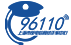﻿ 日黄金当月连续(JAUC)_行情中心_东方财富网
• 上证- - -(涨:-平:- 跌:-)深证- - -(涨:-平:- 跌:-)

-

- -

• 成交量:-(手)
• 今开:-
• 最高:-
• 涨跌幅:-
• 外盘:-
• 仓差:-
• 持仓量:-(手)
• 昨结:-
• 最低:-
• 涨跌额:-
• 内盘:-
• 日增:-
• 10:23• 主要指标
• 均线
• EXPMA
• BOLL
• SAR
• BBI
• RSI
• KDJ
• MACD
• W&R
• DMI
• BIAS
• OBV
• CCI
• ROC
• CR
• BOLL
• RSI
• KDJ
• MACD
• W&R
• DMI
• BIAS
• OBV
• CCI
• ROC
• CR
• BOLL
 交易品种 - 交易代码 - 市场代码 - 交易所简称 东京商品交易所 交易单位 - 最后交易日说明 - 最小变动价位 - 合约月份说明 -

## 日黄金评论

### 日黄金吧;